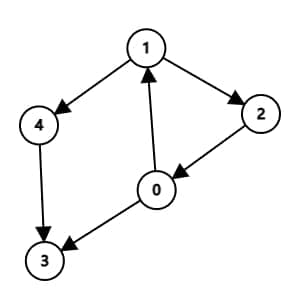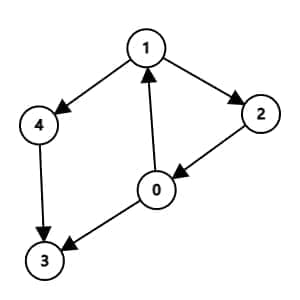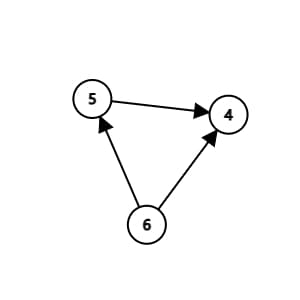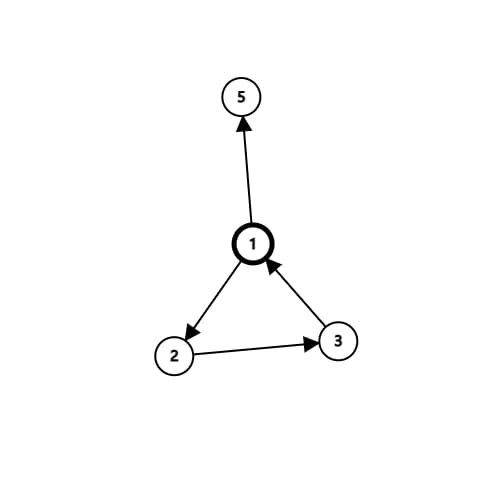## 干啥的

Tarjan是用来求强连通分量的算法tarjan算法的过程大概就是DFS，上代码！

  1 2 3 4 5 6 7 8 9 10 11 12 13 14 15 16  void tarjan(int x){// low[x]=dfn[x]=++depth; vis[x]=1; q.push(x); Graph(i,x) if(!dfn[e[i].v])tarjan(e[i].v),low[x]=min(low[x],low[e[i].v]); else if(vis[e[i].v])low[x]=min(low[x],low[e[i].v]); if(dfn[x]==low[x]) while(y=q.top()){//缩点main(找到了强连通分量，加入一个点中) q.pop(); sd[y]=x; vis[y]=0; if(x==y)break; a[x]+=a[y]; } } 

## 有啥用

### 1.缩点### 2.割点P3387 【模板】缩点

P3388 【模板】割点（割顶）

Graph Editor

Built with Hugo